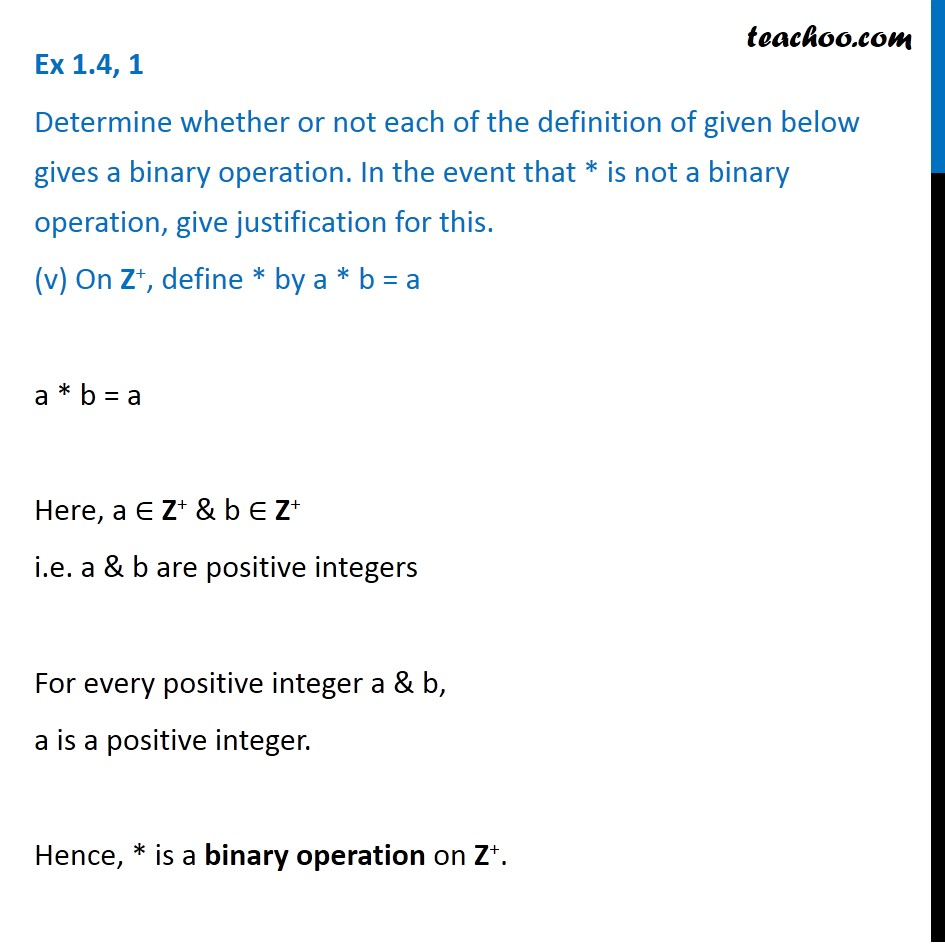1. Chapter 1 Class 12 Relation and Functions (Term 1)
2. Serial order wise
3. Ex 1.4

Transcript

Ex 1.4, 1 Determine whether or not each of the definition of given below gives a binary operation. In the event that * is not a binary operation, give justification for this. (v) On Z+, define * by a * b = a a * b = a Here, a ∈ Z+ & b ∈ Z+ i.e. a & b are positive integers For every positive integer a & b, a is a positive integer. Hence, * is a binary operation on Z+.